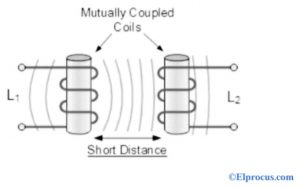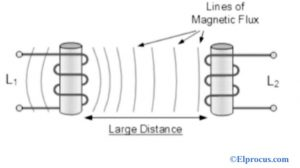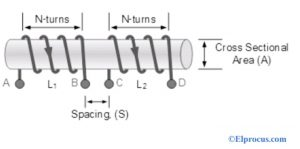# 两个线圈的互感

#### 两个线圈的互感M12 = (N2φ12) / I1

M21= (N1φ21) / I2

M21=第二线圈到第一线圈的互感

N2=第二个线圈的匝数

N1=第一个线圈的匝数

I1=流过第一个线圈的电流

I2=流过第二个线圈的电流。

L1 = (μ0.μr.N1 2 .A) / L

L2 = (μ0.μr.N 2 .A) / L

2 = L1。L2

M=√(L1.L2) 亨利

L1 和 L2 之间 100% 磁耦合